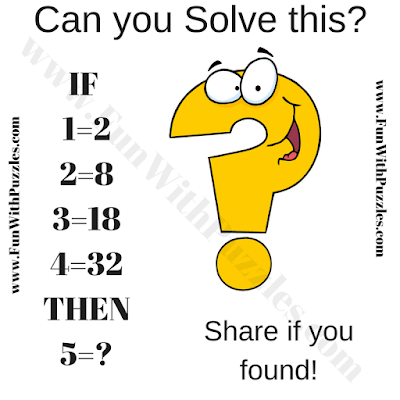This is a Logical Easy Math Question for Teens. In this Logical Picture Puzzle, there are some number equations. These equations denote some logical relationship between the given numbers. Can you find this logical relationship between the given number and then find the missing number which replaces the question mark?Can you solve this Logical Easy Math Question?

The answer to this "Easy and Logic Math Question", can be viewed by clicking on the button. Please do give your best try before looking at the answer.